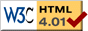# Colliding Blocks

previous next
• Sim
• Graph
• Time Graph
• Multi Graph

This simulation shows two blocks moving along a track and colliding with each other and the walls. One spring is attached to the wall with a spring. Try changing the mass of the blocks to see if the collisions happen correctly.

You can change parameters such as mass, spring stiffness, and friction (damping). You can drag either block with your mouse to change the starting positions.

The math behind the simulation is shown below. Also available are: open source code, documentation and a simple-compiled version which is more customizable.

## Collisions and Conservation of Momentum

The spring and block on the left use the same model as the single spring simulation. For a collision with the wall, we simply reverse the velocity. For collisions between moving blocks we use the law of conservation of momentum to determine the new velocities.

Define the following variables:

• v1i, v2i = initial (pre-collision) velocity of blocks
• v1f, v2f = final (post-collision) velocity of blocks
• vcm = velocity of center of mass
• v1cm, v2cm = velocity of blocks in center of mass frame
• m1, m2 = mass of blocks
• pi, pf = initial and final momentum of system of blocks

We find the velocity of the center of mass of the 2-block system.

 vcm = m1 v1i + m2 v2i m1 + m2

Next we find the velocity of each block in the coordinate system (frame) that is moving along with the center of mass.

v1cm = v1ivcm
v2cm = v2ivcm

Next we reflect (reverse) each velocity in this center of mass frame, and translate back to the stationary coordinate system.

v1f = −(v1ivcm) + vcm
v2f = −(v2ivcm) + vcm

If you fully expand the above you get

 v1f = −v1i + 2(m1 v1i + m2 v2i) m1 + m2
(1)

 v2f = −v2i + 2(m1 v1i + m2 v2i) m1 + m2
(2)

As a check, we can calculate the pre- and post- collision momentum, which should be the same.

pi = m1 v1i + m2 v2i
pf = m1 v1f + m2 v2f

If you expand pf using equations (1) and (2) and simplify you will see that pf = pi as expected.

## Handling Collisions in Softwaresimulation of two balls colliding

Simulations on the computer are discrete in the sense that time advances in "chunks", not smoothly. In the diagram, we calculated the state of the world at time t = 10.0 and then at time t = 10.1 . But the collision happened sometime inbetween. So by the time we detect a collision, the objects are overlapping each other -- a physically impossible state.

The simulations on this website handle collisions as follows:

• Detect that a collision occurred
• Re-run the simulation to very close to (but just before) the time of collision. The time of collision is found by a binary search process.
• Handle the collision by modifying the state of the simulation, such as fixing the velocities as described above.
• Continue to run the simulation up to the current 'now' time. If additional collisions happen they are handled in the same way.

If you are not running the simulation in real time, you can take as long as you want to get as much accuracy as desired. But for a real time simulation, you may need to accept lower accuracy or use some fancier programming. For example, instead of using trial and error to find the time of collision, you could try to predict the time of collision based on the state of the system.

Other examples of simulations with collision handling are Roller Coaster with Flight, Molecule 2 and Rigid Body Collisions.

This web page was first published April 2001.

previous next